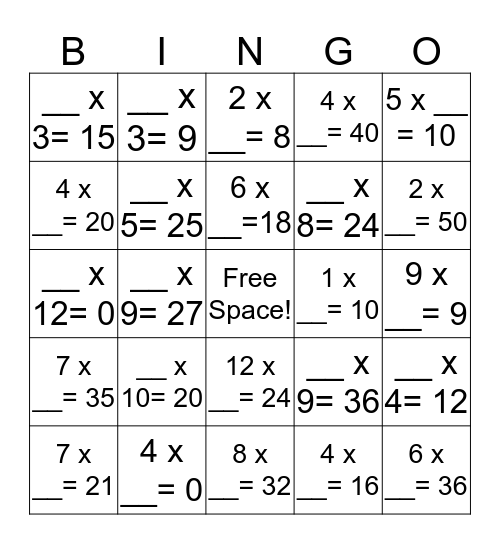# Skittles BINGOThis bingo card has a free space and 29 words: 1 x __ = 9, 4 x __= 0, 2 x __= 8, 3 x __= 12, 5 x __ = 10, __ x 3= 15, __ x 5= 25, 6 x __=18, 1 x __= 10, __ x 4= 12, 7 x __= 21, __ x 9= 27, 4 x __= 16, 6 x __= 36, __ x 12= 0, 3 x __= 30, 8 x __= 32, __ x 9= 36, 2 x __= 50, 7 x __= 35, __ x 10= 20, 8 x __= 48, __ x 8= 24, 9 x __= 9, 4 x __= 20, __ x 3= 9, 12 x __= 24, 4 x __= 40 and 10 x __= 20.

## Play Online